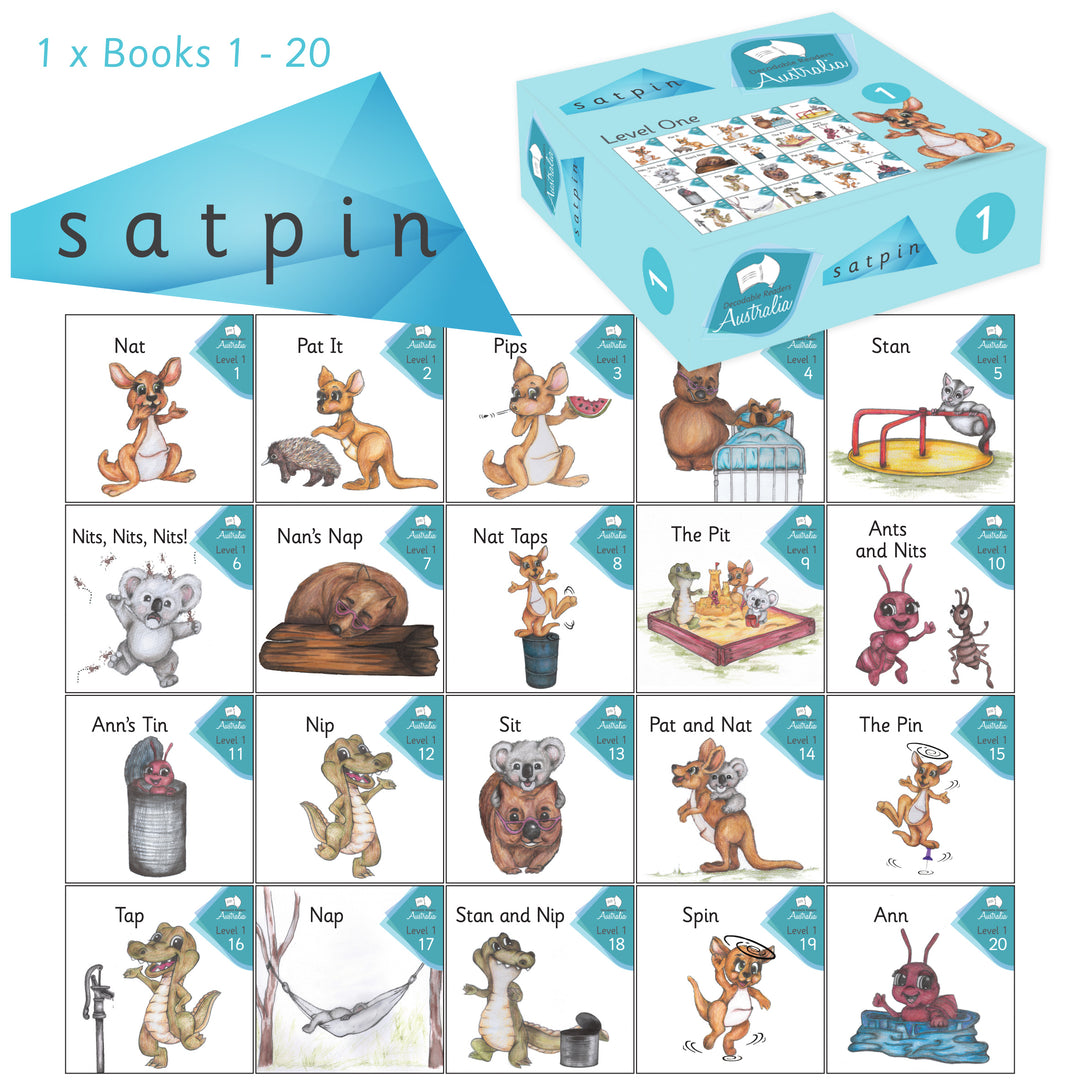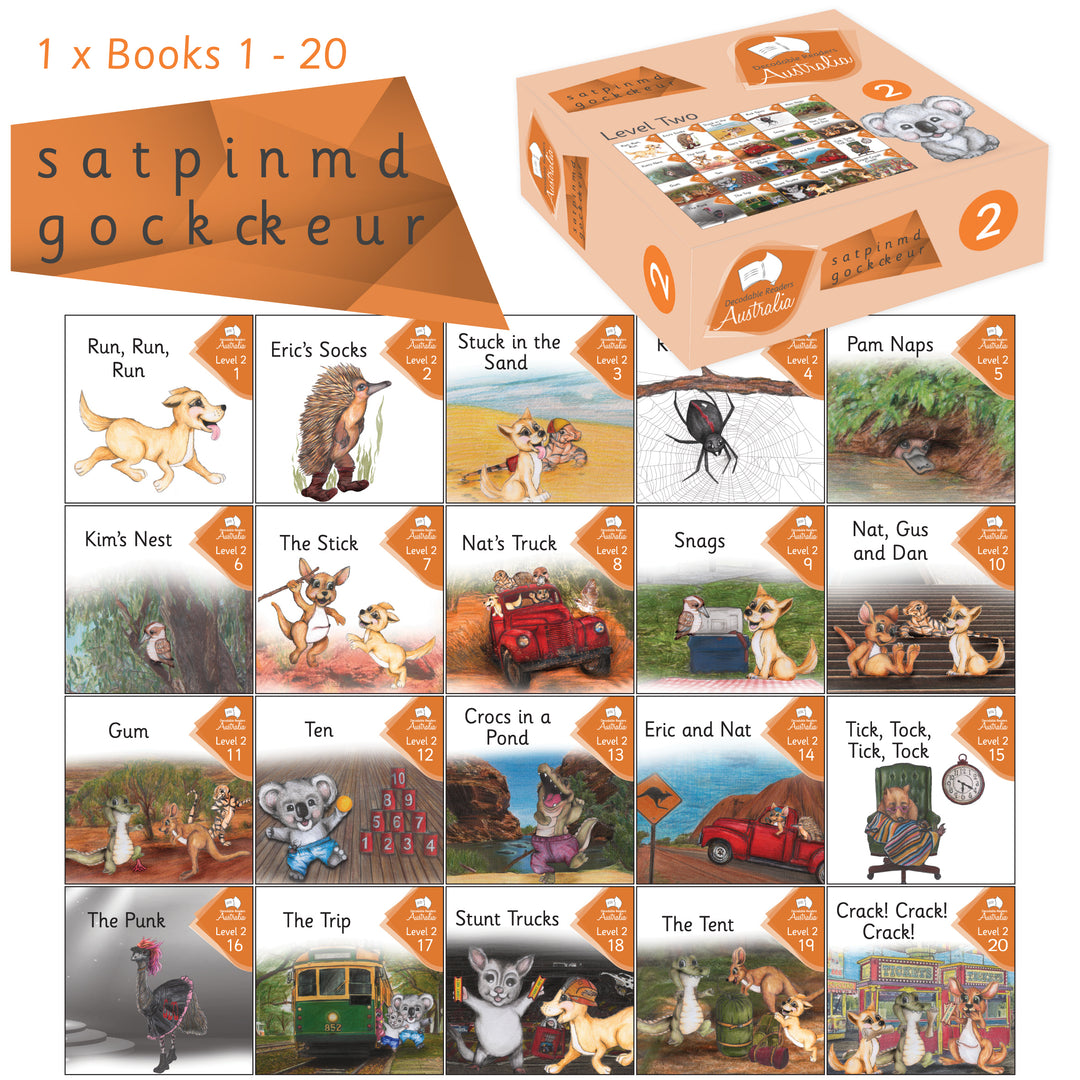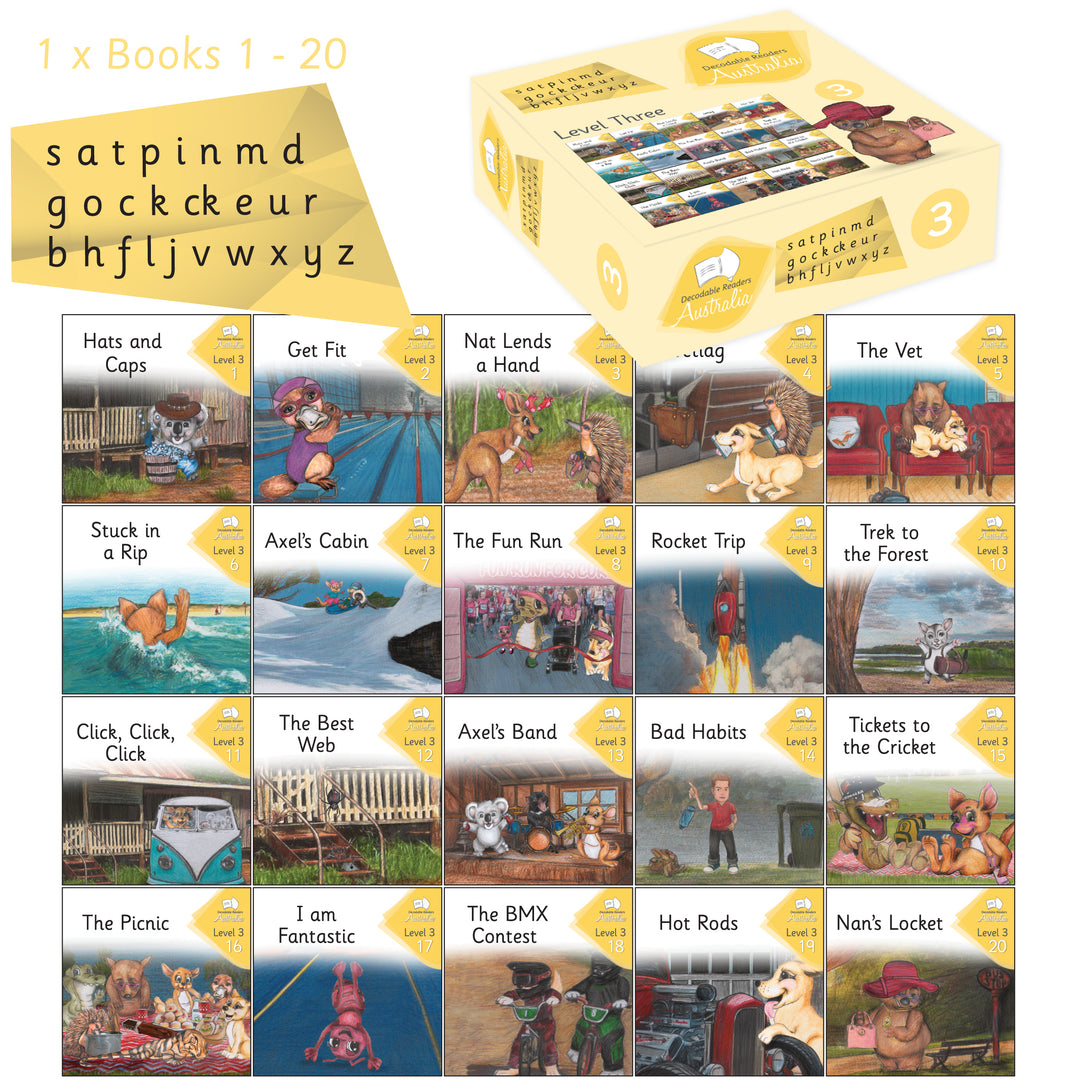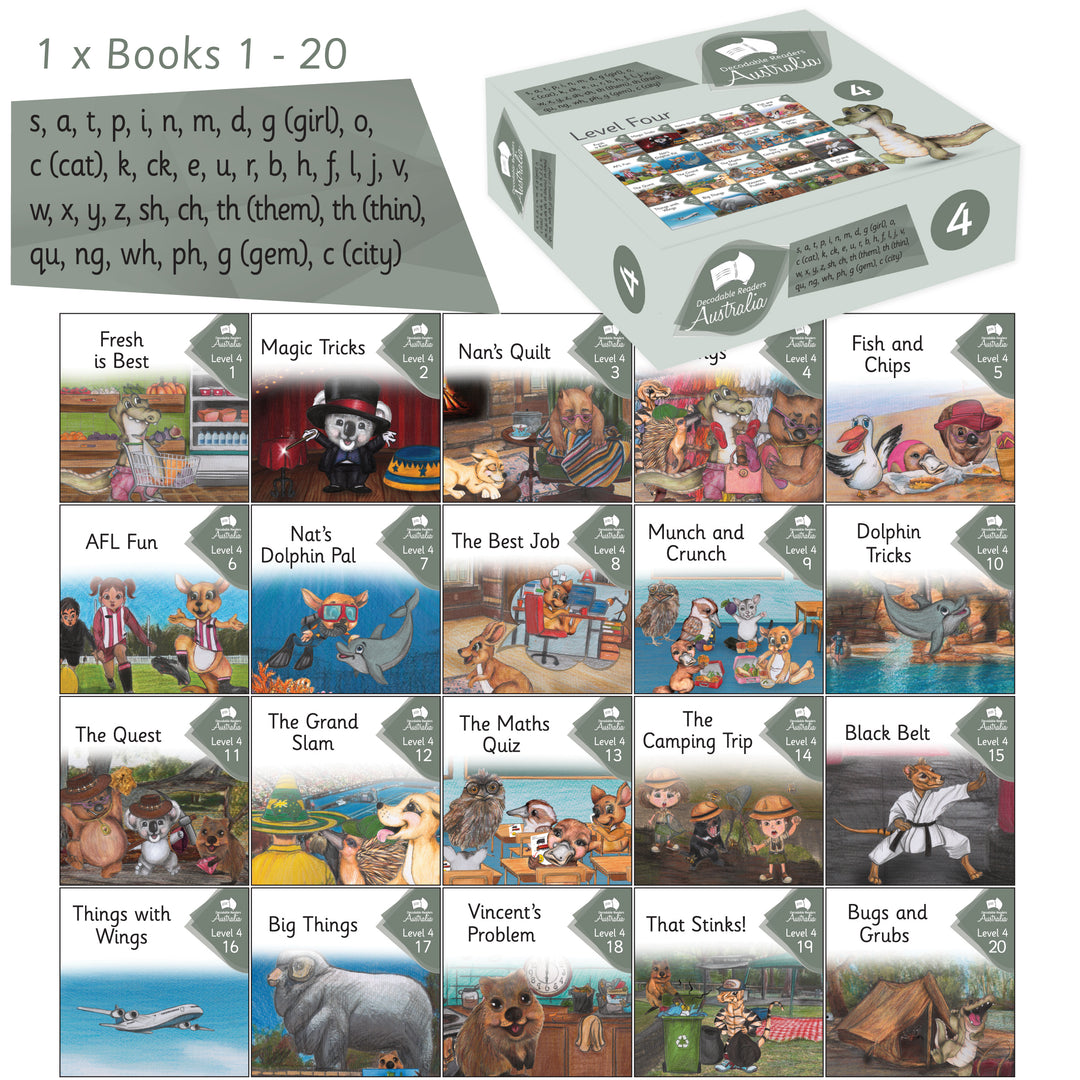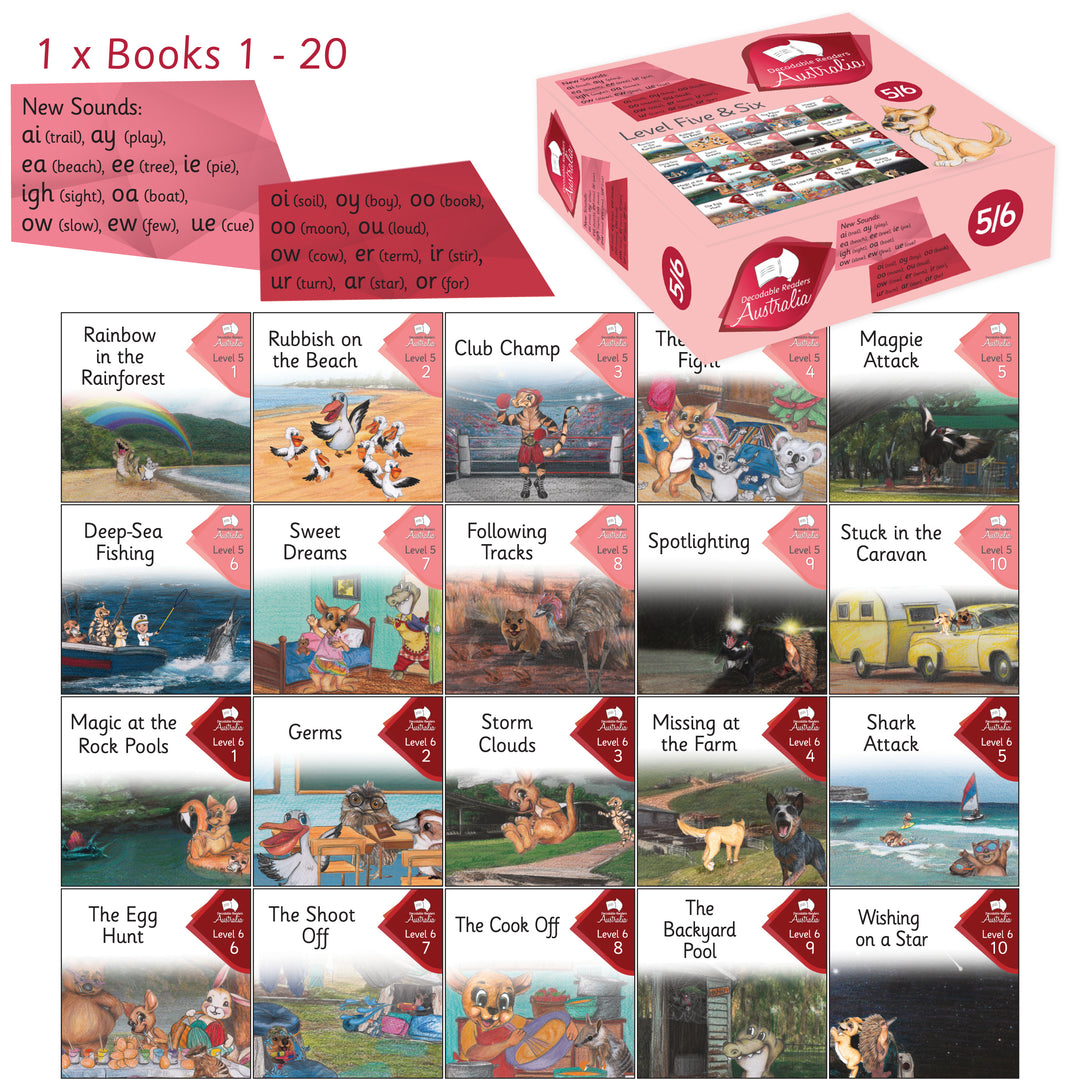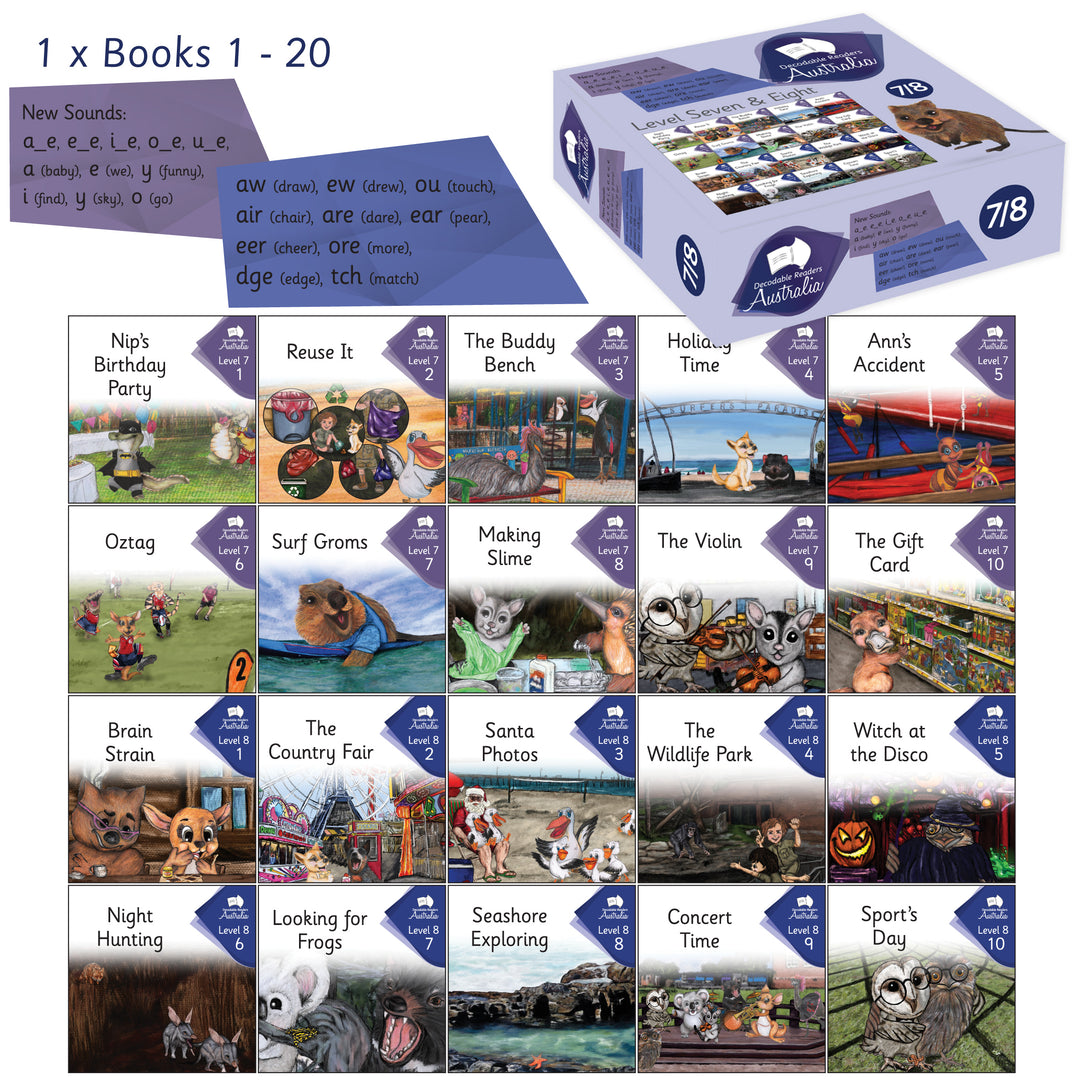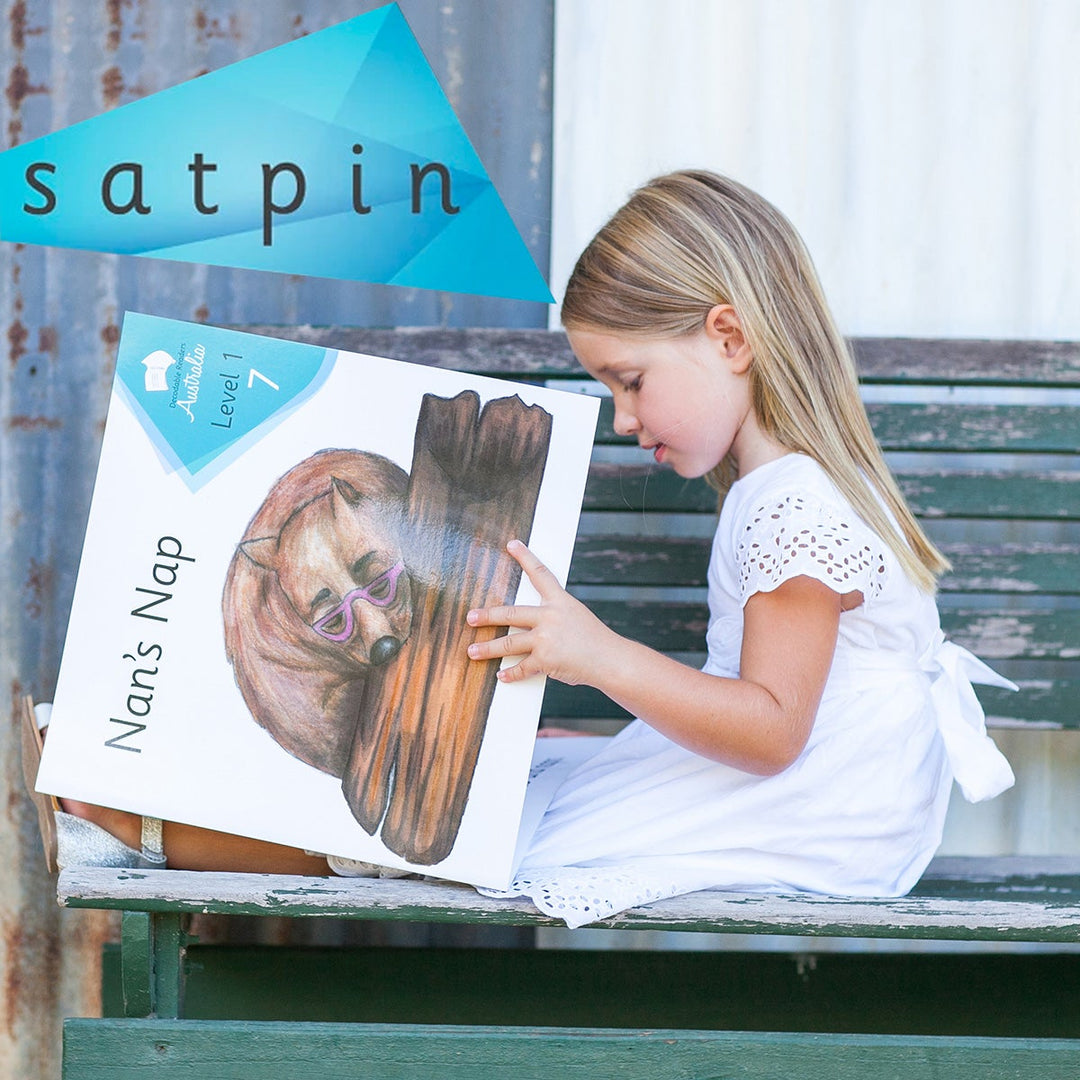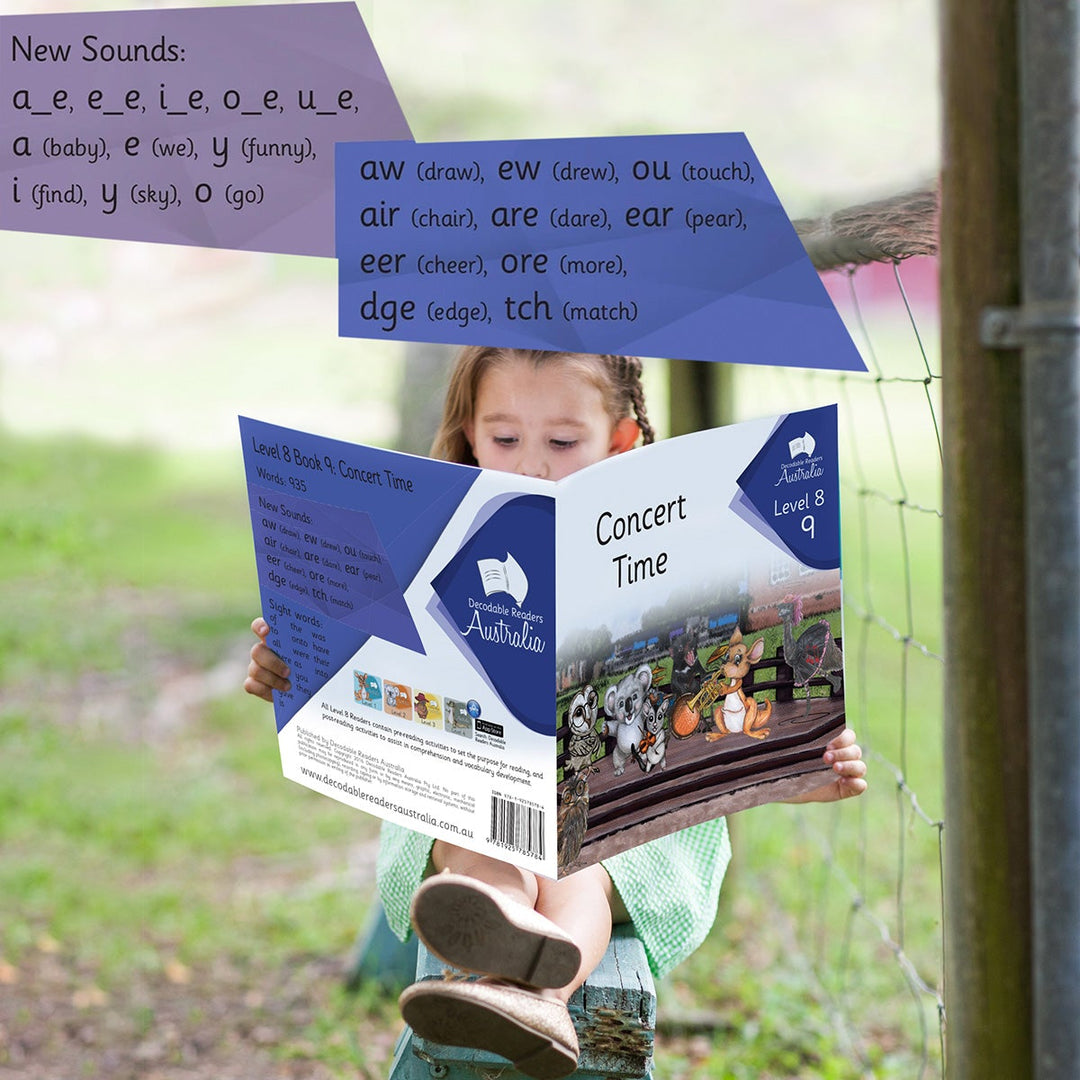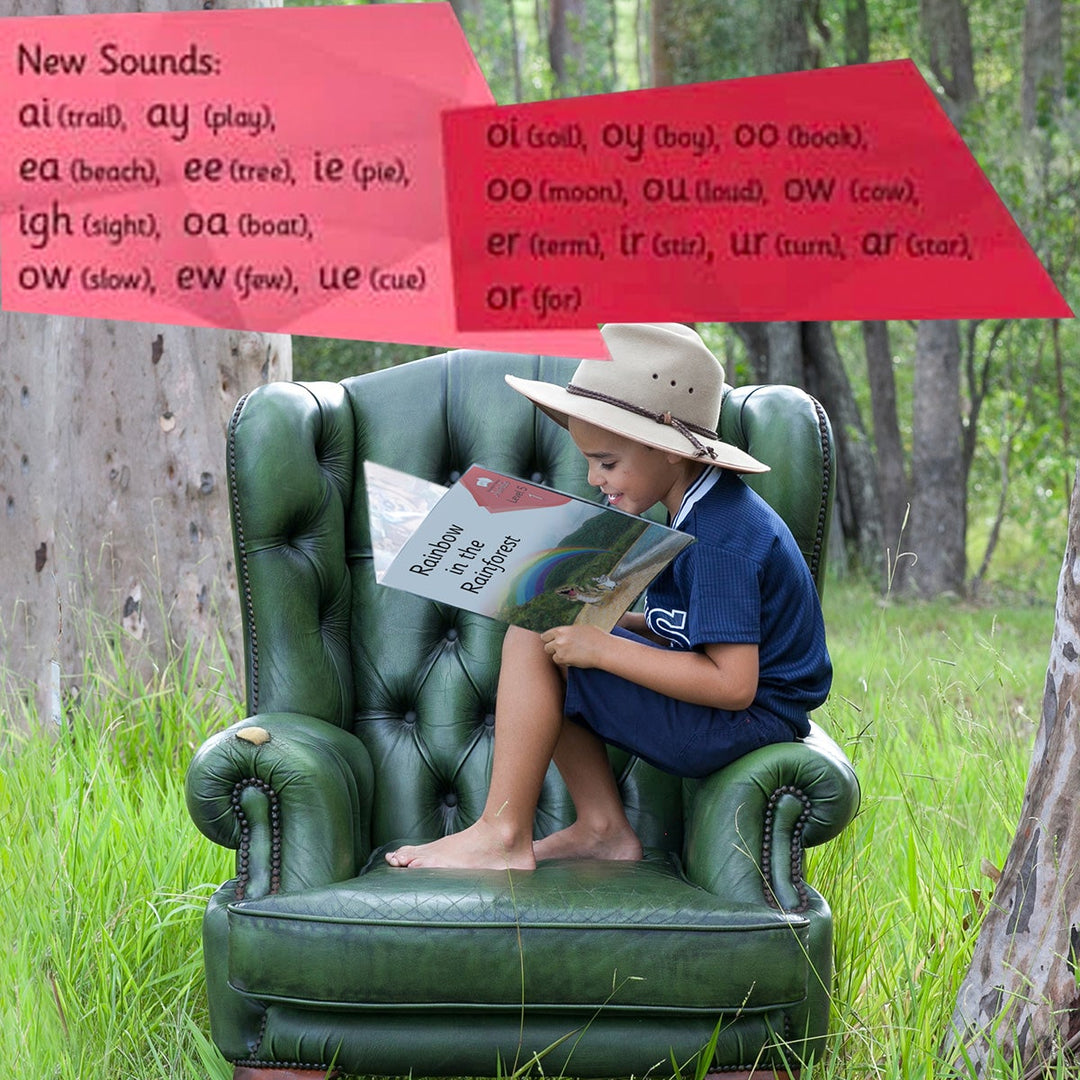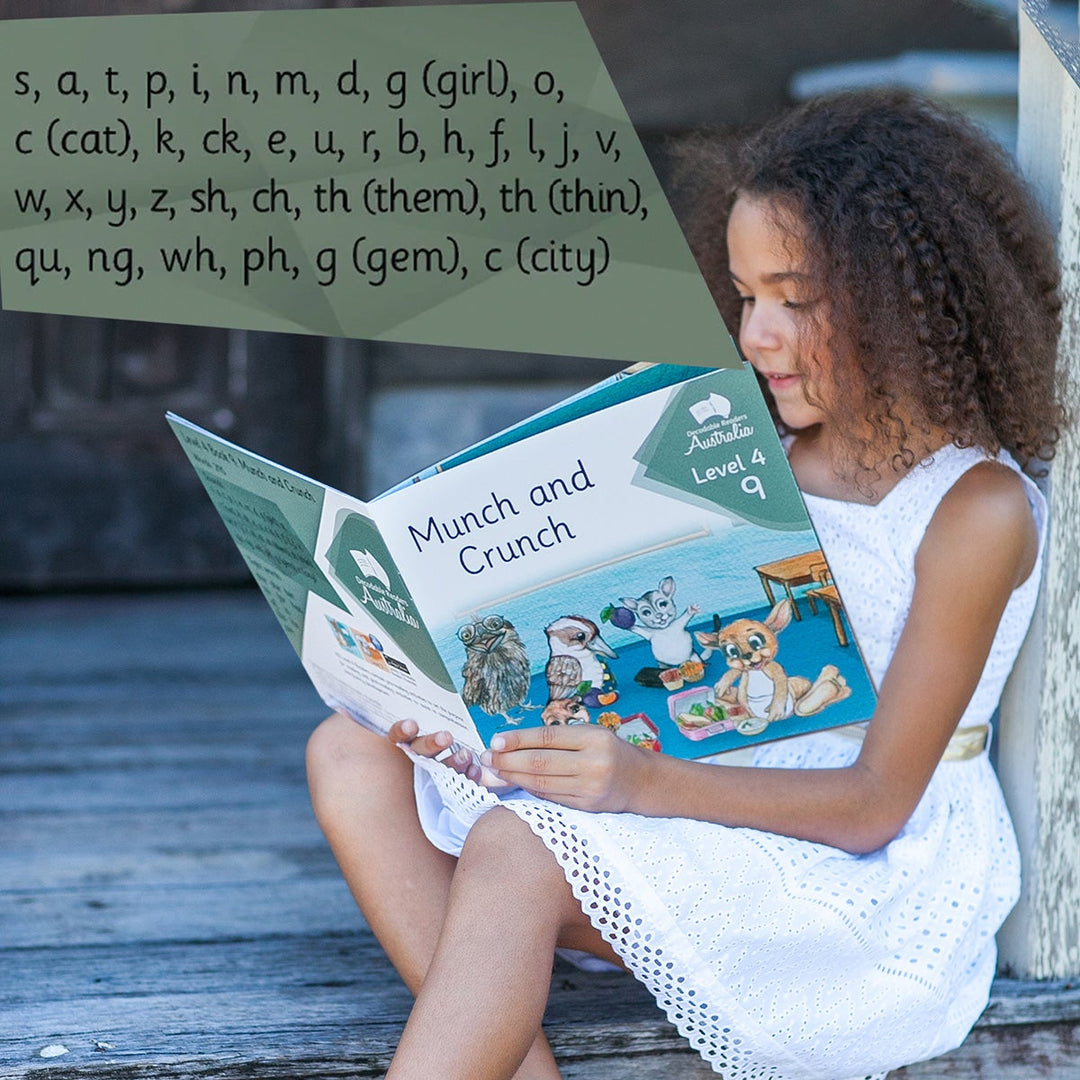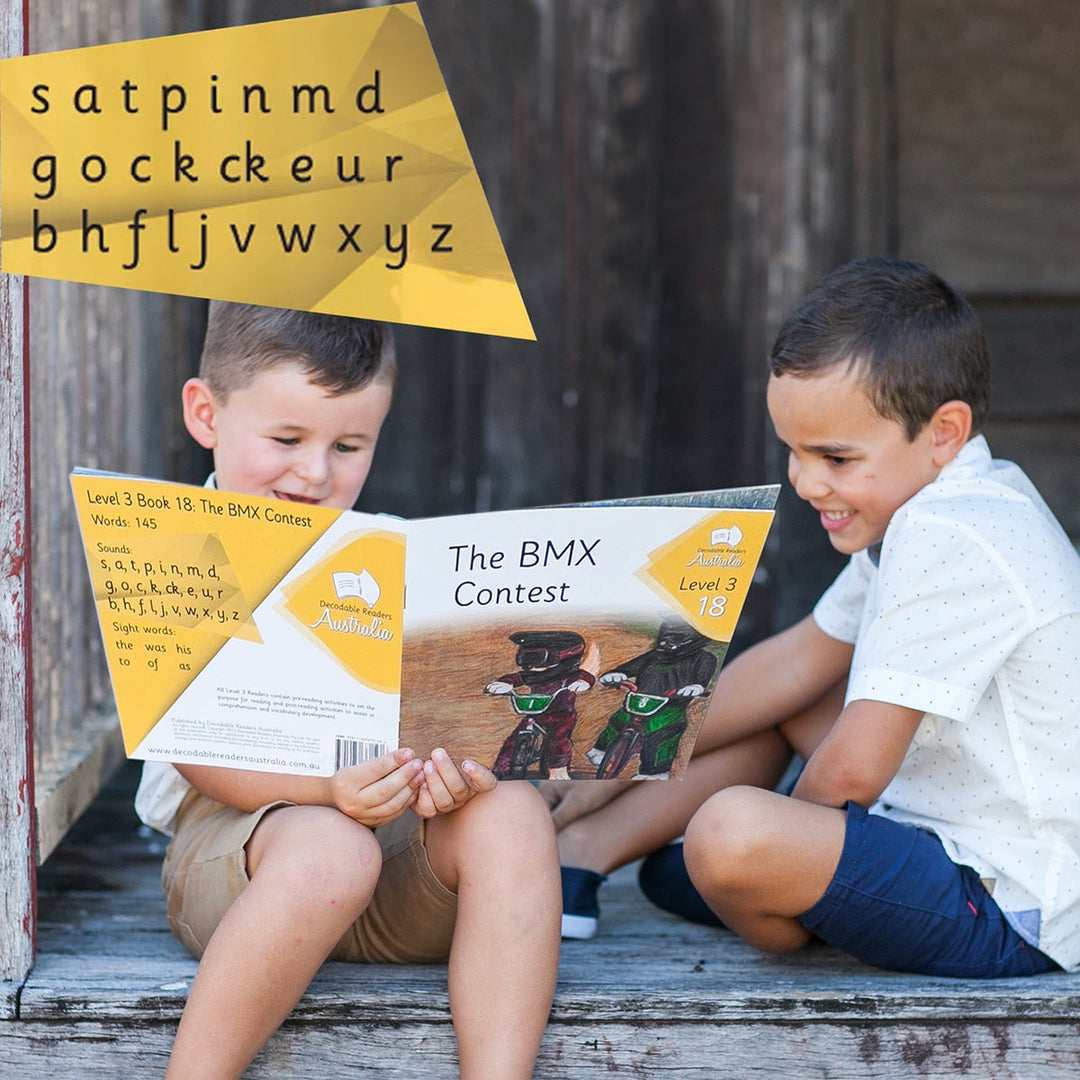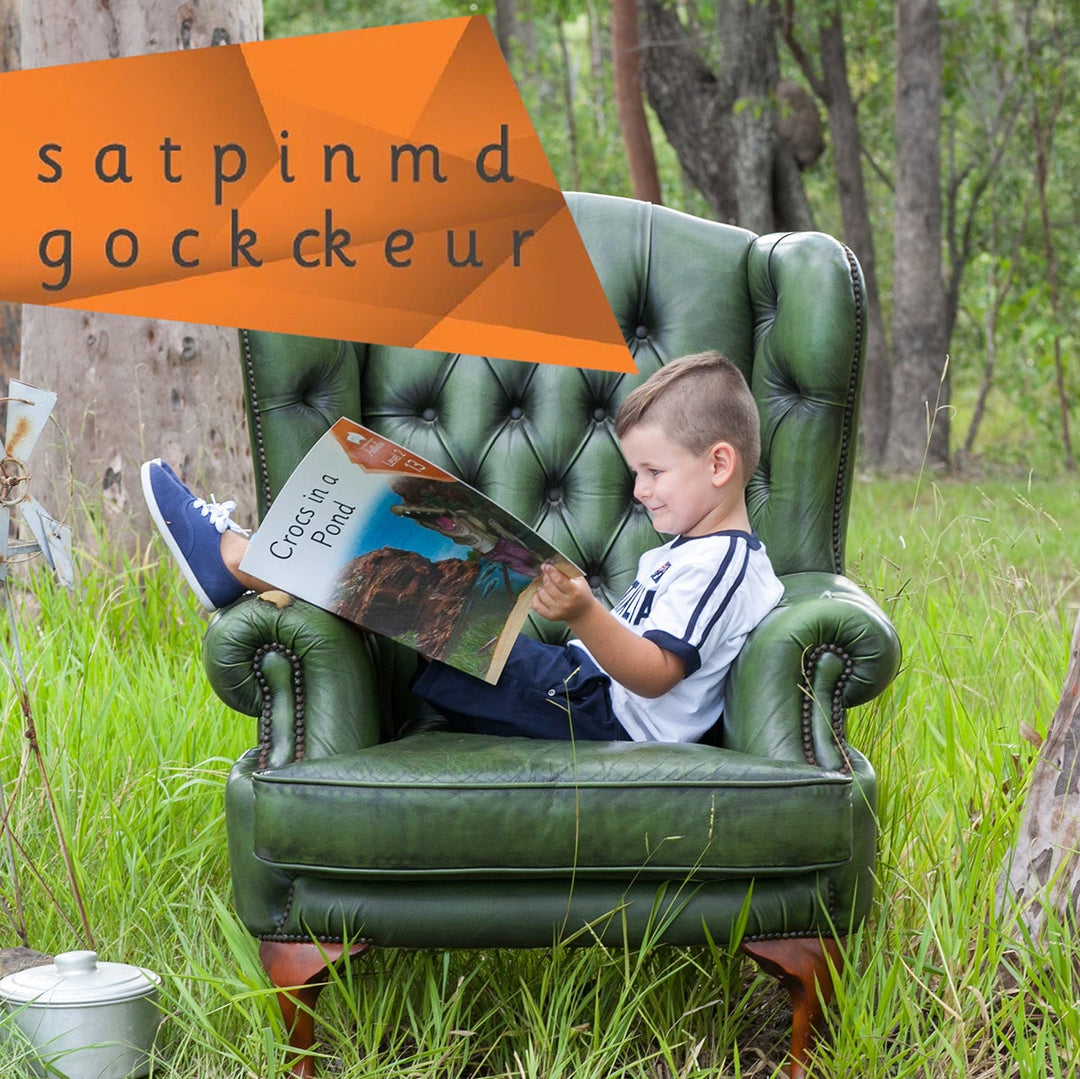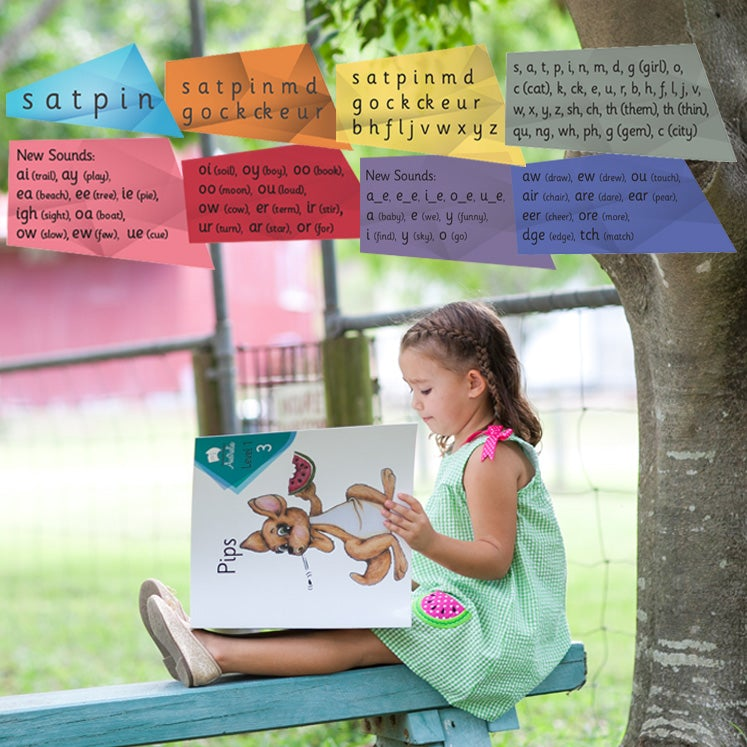# Bulk Resource Pack 1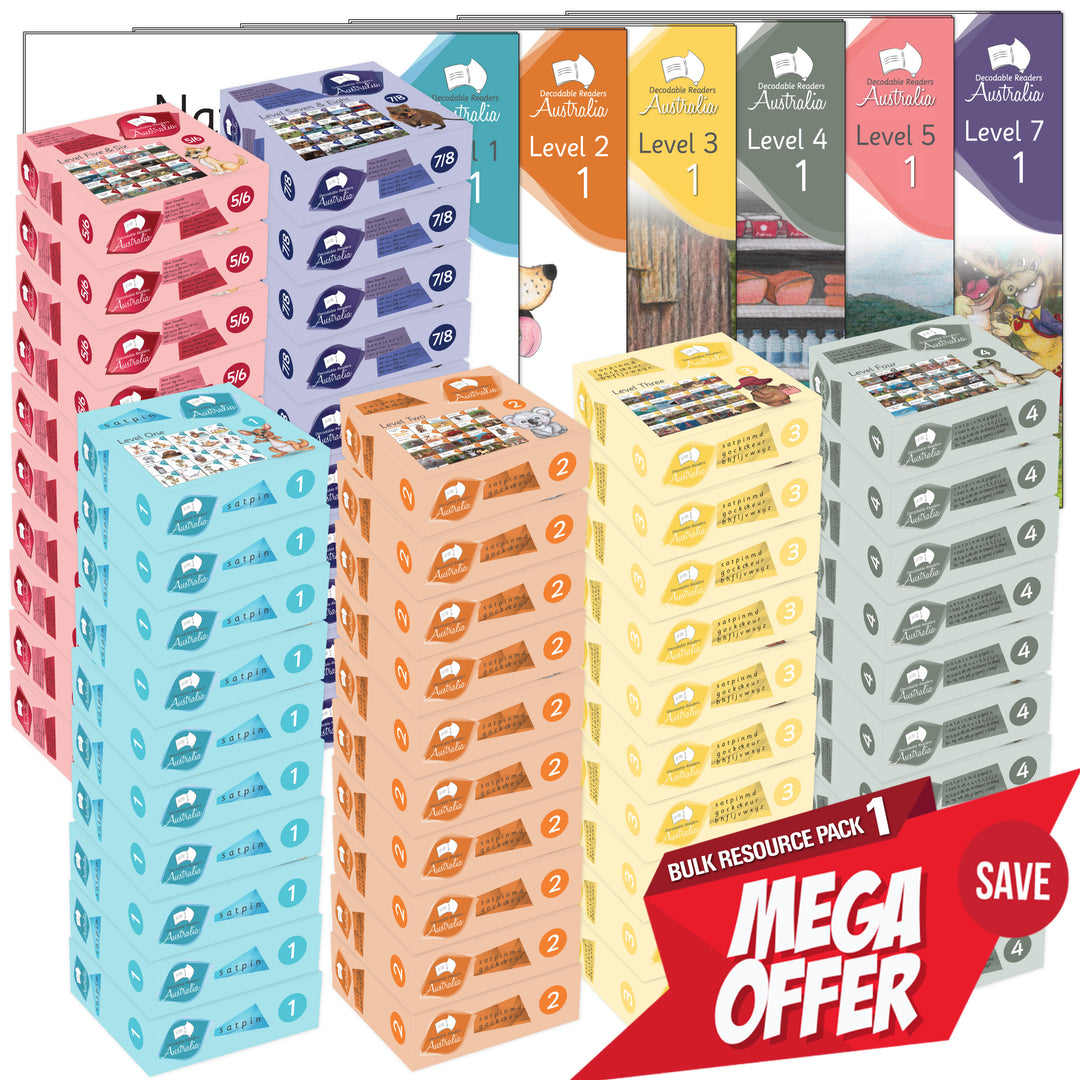\$7,995.00 AUD

\$9,276.00 AUD

Bulk Resource Pack 1
Main Fiction Series Levels 1 to 8

Resource your Early Years Classrooms, Learning Support and Library!

School Bundle – Combined Levels
10x Level 1 = 200 Books
10x Level 2 = 200 Books
10x Level 3 = 200 Books
10x Level 4 = 200 Books
10x Level 5/6 = 200 Books
10x Level 7/8 = 200 Books
= 1,200 Books

+ Complete Big Books Set =
1x Level 1 = 20 Big Books
1x Level 2 = 20 Big Books
1x Level 3 = 20 Big Books
1x Level 4 = 20 Big Books
1x Level 5/6 = 20 Big Books
1x Level 7/8 = 20 Big Books
= 120 Big Books